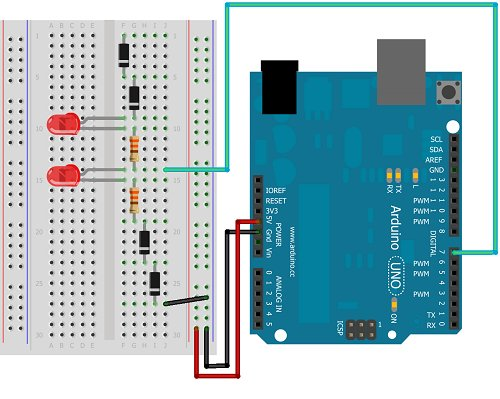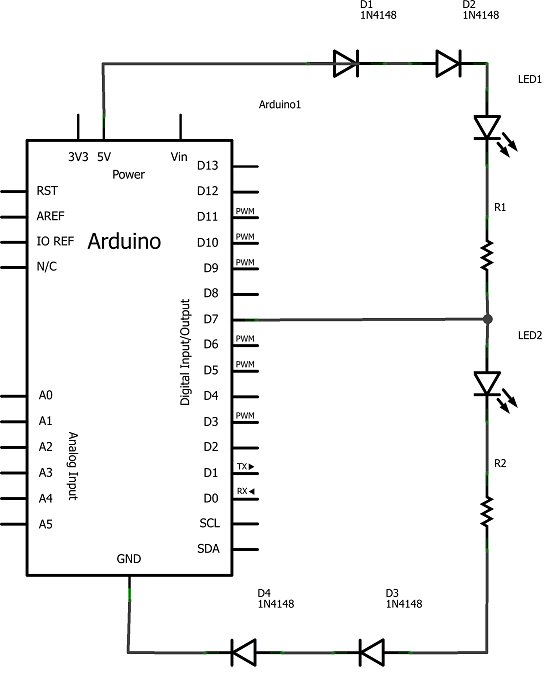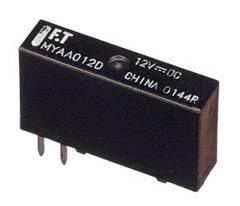# Tri-State Logic

## Introduction

So far we have sent one of two values to any Arduino output pin, either HIGH or LOW. This project shows how we can exploit a third state of the Arduino pins to reduce the number of pins needed to control LEDs. In the project, we’ll control 2 LEDs using only one Arduino pin.

## You Will Need

• 4 x Diodes
• 2 x LEDs
• 2 x 330 Ohm Resistors
• Jumper Wires

Diodes have to be the correct way around to function. Current passes through the diode in the direction of the thin black line on the diode.## Making The Circuit

The following diagram shows how to wire this circuit.

The following diagram shows the schematic for the circuit, which may help you to understand how this process works,

If pin 7 is set to output LOW, LED 1 is lit. If pin 7 is set to output HIGH, there is no drop in voltage across the first LED and so only the second LED is lit. By rapidly switching the output from HIGH to LOW, both pins appear to be lit at the same time. This is due to the what is commonly referred to as the persistence of vision effect. There are some myths about how and why this effect occurs and several explanations are possible. Regardless, if the switching is quick enough, it looks like both LEDs are on at the same time. In order to switch both of the LEDs off, pin 7 is turned into an input pin.

The diodes in this circuit are used to drop the voltage in the circuit, if only 2 are used (one at each end of the circuit), the LEDs remain dimly lit. Although there are 2 resistors in the circuit, only one is needed since the LEDs are never actually on at the same time.

## Programming The Arduino

The following sketch contains the code needed to turn each LED on individually, both at the same time, and to turn them both off,

`int tripin = 7;`

void setup()
{
pinMode(tripin, OUTPUT);
}void loop()
{
pinMode(tripin, OUTPUT);
digitalWrite(tripin, HIGH);
delay(1000);
digitalWrite(tripin, LOW);
delay(1000);
bothOn();
bothOff();
delay(1000);
}

void bothOn()
{
for (int i =0;i<1000;i++)
{
digitalWrite(tripin, HIGH);
delay(1);
digitalWrite(tripin, LOW);
delay(1);
}

}

void bothOff()
{

pinMode(tripin, INPUT);
}

For more detail: Tri-State Logic

#### This Post / Project can also be found using search terms:

• arduino tristate pins
• tristate logging on arduino
• arduino tri state output
• zrduino mega tristate